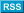HomeResearchResearchDepartment of Complex Analysis and Differential Equations Български
 Faculty of Mathematics and Informatics - Department of Complex Analysis and Differential EquationsDepartment of Complex Analysis and Differential Equations Research directions     Distribution of the zeros of polynomials and entire functions. Orthogonal polynomials Extremal problems in the Approximation theory - theoretical research and computation Local theory of the analytic functions. Uniform coverings Convergence of the method of finite difference applied to the solution of the initial value problem and to the boundary value problem for functional - differential neutral type equations and integro-differential equations Geometry of Lie groups and applicationsto thelinear systems of differential Uniform coverings of topological spaces Analytic number theory - additive problems involving primes, applications of the Hardy-Littlewood circle method, sieve methods, estimates of exponential sums over primes and the distribution of the zeros of the Dirichlet L-functions Connections and metrics on a manifold with a semi-tangential structure Oscillatory and asymptotic properties of the solutions of functional-differential equations of neutral type                                                              Chair:  Assoc. Prof. Ivanka Kasandrova, PhD
 More...